SYLLABUS  Previous: 3.1 Option pricing for  Up: 3 FORECASTING WITH UNCERTAINTY  Next: 3.3 Improved model using

3.2 Simple valuation model using binomial trees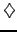[ SLIDE tree - delta hedging - transition probability - matching volatility - recipe || VIDEO modem - LAN - DSL ]

A single step binomial forecast provides only a crude approximation for the fair price of an option before it expires. To increase the accuracy of the model, an obvious improvement would be to extend the number of possible outcomes; the evolution of the forecast price could also be modeled more accurately by allowing them to reverse trends during the calculation. Both can be achieved by dividing the lifetime of the option [0;T]   into a number smaller time intervals of duration Dt   and performing the calculation recursively with the binomial tree model sketched in (3.2#fig.1). In addition, we show here how a tree is constructed to reproduce log-normal price increments that are typical for stock: rather than adding / subtracting a constant as in the previous sect.3.1 (for a normal distribution), possible realizations are here obtained by multiplying / dividing by a constant factor (for a log-normal distribution).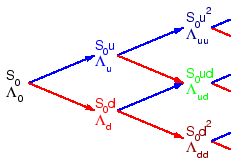For each step starting with the present value of the underlying S0  two new forcasts are obtained by multiplying the value on each node by the factors u or d  to mimic possible movements up or down until the entire lifetime of the option is covered by the tree. A perfectly hedged portfolio is then constructed starting from every branching point closest to the expiry date (work backwards from the right of (3.2#fig.1), by combining an amount delta of the underlying with a (conventionally short) position of a (positively correlated) option. By demanding that the portfolio be risk free, the movement up or down produce the same return and a new value is obtained for delta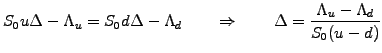(3.2#eq.1)

Since a perfectly hedged portfolio carries no risk at all, the standard no-arbitrage argument shows that it can be discounted back one step in time using the risk free interest rate r  This discounted value (3.2#eq.2, left hand side) has to be equal to the cost of setting up the portfolio before the step is taken (right hand side):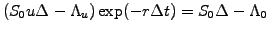(3.2#eq.2)

Substituting the hedging factor delta (3.2#eq.1) and rearranging the terms, this yields an expression to calculate the fair value of an option one step back at a time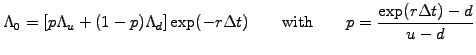(3.2#eq.3)

The parameter p  can be interpreted as the probability of the forecast price moving up and (1-p)  the probability that it will move down in the tree. The scaling factors (u,d)  control the amplitude of the change and have to be carefully chosen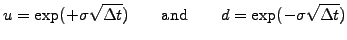(3.2#eq.4)

to reproduce the drift and the volatility observed in the real markets (quants read below).
Although the importance will only appear later, simply note here that the expected value of the underlying calculated using the probability (3.2#eq.3)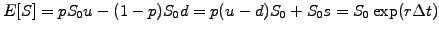(3.2#eq.5)

grows, on average, exactly at the risk free interest rate. Using the probability (3.2#eq.3) therefore implies that the return on the underlying stock is equal to the risk free rate m=r.

Quants: matching the parameters (u,d) with drift and volatility.
For clarity, distinguish the probability of a price moving up in the tree p  from the probability of the price moving up in the real world q.  In the presence of drift, the real world price of the underlying grows exponentially (3.2#eq.6, left hand side), which should be reproduced by the expectation E[S]  from the price forecast in the tree (right hand side):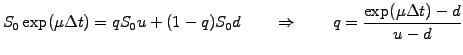(3.2#eq.6)

In the same manner, the real world variance (square of volatility, left) has to be matched with the variance Var[S]=E[S2]-E[S]2  from the price forecast in the tree (right):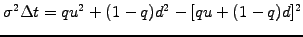(3.2#eq.7)

Substitute the real world probability (3.2#eq.6) into (3.2#eq.7)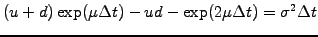(3.2#eq.8)

and expand to first order in the small time steps by writing exp(mD)~ 1 +mD.  The symmetric solution u=1/d  is generally chosen and is the one given in (3.2#eq.4).

To summarize, calculations using binomial trees for the stockmarket can be organized as follows
1. Divide the entire lifetime of the option into a finite number of steps N, ranging from only a few (by hand) up to 30 (using a computer to evaluate 31 possible outcomes that are connected with 230~  1 billion possible paths).
2. Forecast the underlying forward in time (trunk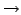leaves), choosing (u,d) according to (3.2#eq.4) to reproduce the historical volatility observed in a real market.
3. Work backward in time (trunk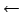leaves) starting from the terminal option payoff; for every neighboring branching point, calculate the hedging delta (3.2#eq.1) and the option price at the previous time step (3.2#eq.3). In the case of American options, substitute the (larger) intrinsic value that can be obtained from an early exercise when the calculated price drops below this intrinsic value.
4. The final result is obtained on the trunk of the tree and is an approximation of the fair value of the option before the expiry date, with an accuracy proportional to 230.
Because it involves only simple mathematics, binomial trees are ideally suited to develop an intuition for option pricing (exercise 3.01, 3.02). Some practitioners even use trees to evaluate option prices with a computer: the forthcoming sections will show that differential calculus provides a far better framework to account for the features appearing in exotic contracts. Indeed, without having to account for these features, the computer is not really needed: the price can simply be calculated from an analytic solution of the Black-Scholes differential equation, which we are about to derive.

SYLLABUS  Previous: 3.1 Option pricing for  Up: 3 FORECASTING WITH UNCERTAINTY  Next: 3.3 Improved model using